### a. Principle of Reversion

The Principle of Reversion is a methodology by which instrumental error can be determined. By reversing the geometry of a given instrument condition, we can see twice the error present in that condition. Then either by procedure, adjustment, or computation, the error can be compensated. Because they can be compensated, errors resulting from axes maladjustment are systematic.
We will see how reversion can be used to procedurally compensate axes maladjustment when setting up and using the instrument.

### b. ABT not perpendicular to VA

If these axes are perpendicular then when the tube bubble is centered (ABT 1) and the instrument is rotated 180° (ABT 2) the bubble will stay centered, Figure B-3.Figure B-3 Bubble Reversion

If the two axes are not perpendicular, then when the bubble is centered in the first position the VA is not vertical, Figure B- 4.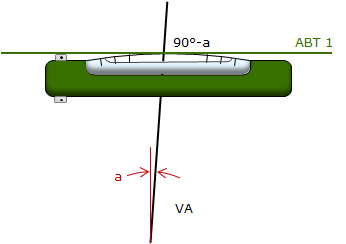Figure B-4 Bubble Maladjustment

The angle between the VA and the true vertical is the angle "a" and is the same angle error in the non-perpendicular condition.

Because the VA is the TSI's rotational axis and the ABT is fixed with respect to it, when the instrument is rotated 180° we have the situation in Figure B-5.Figure B-5 Bubble Run

The (90°-a) condition is maintained between the ABT and VA. Rotated it shifts ferom one side of the VA to the other. The total angle change in the ABT orientation is the angle "b". What does b equal? The total angle from one end of ABT 1 to the other is 180°:

180° = b+(90°-a)+(90°-a) = b+90°-a-90°-a
180° = b+180°-2a → b = 2a

The angle b is twice the actual error a. That means when the bubble runs, it runs twice the error amount. If we bring it back halfway, we compensate the non-perpendicular condition, Figure B-6: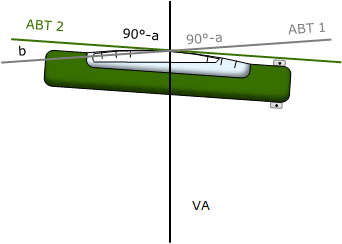Figure B-6 Compensating Bubble Run

If the bubble runs three divisions, it is brought back one and a half divisions using the TSI's leveling screws.

That will make the VA vertical and cause one end of the tube bubble to always be higher by the same amount (eg, 1-1/2 div) as the TSI is rotated.

The bubble is still off, because of the error, but the VA will be vertical.

### c. OPA does not coincide with VA

Most TSIs have their optical plummet built into the instrument base. That means the plummet's eyepiece rotates with the instrument. On older TSIs, and stand alone tribrachs, the eyepiece is built into the tribrach and does not rotate with the instrument. Regardless the design, the purpose of both is the same: to take the place of a plumb bob in locating a TSI over a ground point.

There are two methods to check the OPA-VA coincidence depending on the plummet's integration. Because the optical plummet is a telescope and two images are viewed (ground point and index mark), it is important to clear parallax before beginning.

#### Method (1)

Used when the optical plummet eyepiece rotates with the instrument.

When the TSI is level and the plummet mark on the ground mark, the plummet mark should stay on the ground mark as the TSI is rotated, Figure B-7. This can only be checked after the instrument is accurately level, accounting for any ABT error as described above.Figure B-7 Otical Plummet Check

If the two axes do not coincide the error is angle c, Figure B-8. The VA is vertical, but the OPA isn't, so the ground mark sighted thru the plummet is not vertically beneath the TSI. When the TSI is rotated, the non-coincident condition is moved to the other side of the VA pulling plummet mark off the ground markFigure B-8 Optical Plummet Maladjustment

Because of reversion, the distance the plummet mark moves is twice the error present. The point vertically beneath the instrument is halfway between the two plummet mark locations, Figure B-9.Figure B-9 Effect of Maladjusted Optical Plummet

If you rotate the TSI to various positions and check the optical plummet you would see that its mark scribes a circular path centered on the ground mark, Figure B-10.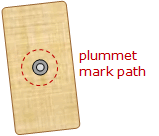Figure B-10 PLummet Path

#### Method (2)

The first method will not work with a fixed optical plummet because its position cannot be changed 180°. Instead, an easy way to check a fixed plummet is to use a plumb bob. Attach the TSI to a tripod that has a plumb bob hanger. After accurately leveling the TSI make sure the plumb bob is centered over the ground mark, Figure B-11. Remove the plumb bob and sight through the optical plummet. If the plummet is in adjustment it will be centered on the mark. If not centered it shows how much the plummet is out of adjustment.Figure B-11 Fixed Optical Plummet Check

Because a plumb bob was used to set up the TSI it is correctly centered over the ground point so it does not have to be moved.

This method can also be used to check a TSI with a rotating optical plummet.

### d. HA not perpendicular to VA

#### (1) Horizontal angle

A horizontal angle is the angle between two vertical planes: one contains the TSI and backsight (BS) point, the second contains the TSI and foresight (FS) point, Figure B-12.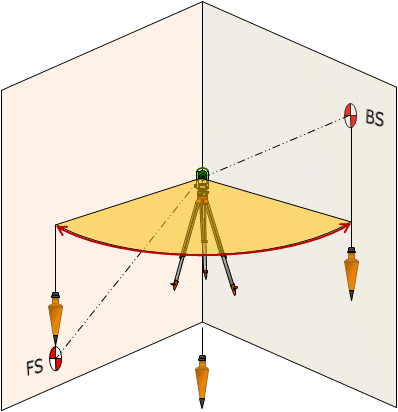Figure B-12 Horizontal Angle

If the BS and FS points are at different elevations, then the telescope must be rotated in a vertical plane during the angle measurement. Because the LoS is constrained to rotate about the HA, if the HA is not horizontal when the TSI is level, then an error is introduced. In Figure B-13, with the HA not horizontal, the LoS moves non-vertically when the telescope is plunged. Instead of sighting point A vertically below point T, the operator sights to its side at point B. The angle between the lines T-A and T-B is the amount of error in the HA.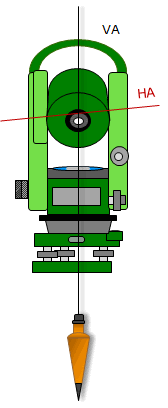Figure B-13 Horizontal Axis Maladjustment Direct Position

Reversing the telescope and rotating the TSI 180° reverses the geometry to that shown in Figure B-14.Figure B-14 Horizontal Axis Maladjustment Reverse Position

In this reverse position, the HA is now inclined in the other direction. As before, sighting the BS target and rotating the telescope downward causes the LoS to follow a non-vertical path, this time to the left. The angle between lines T- C and T-A is the error in the HA and the same as in the direct position.
Because the HA inclination is the same but in opposite direction in the direct and reverse positions, point A on the true vertical line is halfway between points B and C.

#### (2) Vertical angle

Unlike a horizontal angle, reversion does not cancel the instrumental error introduced in a vertical angle when the HA is not perpendicular to the VA. In both the direct and reverse positions, the vertical angle is measure inclined to the vertical, Figure B-15.Figure B-15 Reversing Effect

Both are larger than the true vertical angle. Taking their average does not account for the instrumental error.

### e. LoS not perpendicular to HA

If the LoS is perpendicular to the HA and the HA is horizontal, then revolving the LoS about the HA will create a vertical plane centered on the instrument. If not perpendicular, the LoS sweeps thru a cone whose axis coincides with the HA, Figure B-16.Figure B-16 Line of Sight Maladjustment

This maladjustment can be determined by double-centering which involves revolving the telescope about the HA and rotating the TSI about its VA . The process and effect of LoS maladjustment is described in Figure B-17.

 Top view: Line AB is to be extended to point C. The TSI is set up at B and uses A for a BS.Step (1) The TSI is set up over point B and the LoS pointed at point A in the direct position. If the HA is not perpendicular to the LoS, then it will not be 90° to the BS line.Step (2) The telescope is rotated about the HA to the opposite side placing the TSI in the reverse position.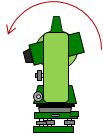Step (3) The non-perpendicular (90°-a) HA/LoS condition is maintained after the rotation. A temporary mark is set.Step (4) The TSI is revolved about the VA keeping the instrument in a reverse position.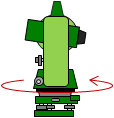Step (5) The HA/LoS non-perpendicular condition is maintained but now the error is on the opposite side. Point A is sighted.Step (6) The telescope is rotated about the HA to the opposite side placing the TSI back in the direct position.Step (7) The non-perpendicular (90°-a) HA/LoS condition is maintained in the rotation. A second temporary mark is set at the same distance as the first.Step (8) The extension of the line AB, point C, is located midway between the two temporary marks.Figure B-17 Double-Centering

### f. Vertical circle index error

#### (1) What is it?

Although a modern TSI uses compensation to minimize index error, it's still worth describing this error in order to understand it.

A traditional non-digital theodolite uses glass circles marked with angular value divisions for its horizontal and vertical circles (think really expensive and accurate protractors). To correctly orient the vertical circle when the instrument is level was done in one of two ways:

• A separate vertical circle bubble, or,
• A compensator which aligned the circle to gravity

In either case, the goal was to align (or index) the circle so that 0° and 180° were along a vertical line while 90° and 270° were along a horizontal line.

Figure B-18 shows a correctly indexed vertical circle used to make direct and reverse readings to a target.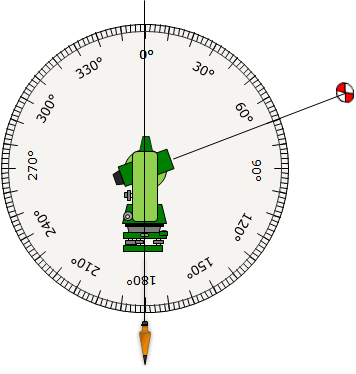Figure B-18 Correctly Indexed Vertical Circle

Direction postion

Zenith angle, Z = 70°
Vertical angle, V = 90°-70° =+20°

Reverse position

Z = 290°
V = 290°-270° = +20°

Reading the angle direct and reverse provides a check on the vertical circle orientation:

• the sum of the two zenith angles, Z, should equal 360°, and,
• both vertical angles, V, should be the same.

If the vertical circle isn't correctly oriented, an index error exists. The incorrect orientation can come from a maladjusted circle bubble or compensator. Figure B-19 shows measurements to the same target but with a vertical circle index error of 4° (this is a very large error, used here only for illustrative purposes).Figure B-19 Vertical Circel Index Error

Measuring only in direct or reverse position alone does not provide sufficient data to determine if index error is present nor how to compensate for it (remember our friend, the Principle of Reversion).

Direct position

Zenith angle, Z = 74°
Vertical angle, V = 90°-74° =+16°

Reverse position

Z = 294°
V = 294°-270° = +24°

Checks?

Sum of the two zenith angles, Z, should equal 360°

Sum = 74° + 294° = 368°
The sum exceeds 360° by 8° which is twice the index error: error = +8°/2 = +4°
The error is subtracted from both the direct and reverse zenith angles.

Both vertical angles should be the same

They aren't but notice the difference between the direct and reverse vertical angles is twice the index error.
That means if we take their average: (16°+24°)/2 = 20° we get the correct vertical angle.
Averaging the direct and reverse vertical angles compensates the error.

Reading the circle in both direct and reverse positions allows the index error to compensate.

#### (2) Single and dual axis compensation

A modern TSI compensation system measures axes tilts and corrects angle measurements accordingly. A particular TSI may use a single axis system which corrects only zenith/vertical angles for axis tilt or a dual-axis system which also corrects horizontal angles errors caused by axes tilt. Either way, index error is compensated by the instrument itself.

That's not to say a TSI's compensation system is infallible. As with any other adjustment aspect of the TSI, the compensation system shouldn't presumed to be without error. While an automatic level's compensator is relatively easy and quick to check, those of a TSI are more complex. The instrument's manual will have information on its compensation system, its sensitivity, and how to check that it is working correctly. Not all TSIs use the same compensation design, even from the same manufacturer, so procedures in the manual should be followed.